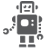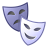## How to calculate i.MX6 IPU CSI capability.

cancel
Showing results for
Did you mean:
SOLVED

## How to calculate i.MX6 IPU CSI capability.

315 ViewsSenior Contributor I

Hi community,

I have a question about i.MX6 IPU CSI.

I want to know how to calculate the CSI capability by the equation in page 2818 in IMX6SDLRM Rev.2.

=====

F = FH * FW * FPS * BI * DF

Where

• FH = frame height (in pixels)

• FW = Frame width (in pixels)

• fps = frame rate (frames per second)

• BI = typically 35% overhead, should be assumed as 1.35. The actual blanking

intervals are a parameter of the attached device.

• DF = data format, defines the number of cycles needed to send a single pixel.

=====

If input 1080p@30 image (YUV422 over 8bit), which calculation is correct?

----------

Calculation A.

F = 1920 pixels * 1080 pixels * 30 fps * 1.35 * 2cycles/pixel

= 167,961,600 Hz

= 168.0 MHz (167,961,600/(1000*1000))

----------

Calculation B.

F = 1920 pixels * 1080 pixels * 30 fps * 1.35 * 2cycles/pixel

= 167,961,600 Hz

= 160.2MHz (167,961,600/(1024*1024))

----------

I think Calculation A is correct, right?

Best Regards,

Satoshi Shimoda

Labels (5)

• ### i.MX6S

Tags (2)
1 Solution
48 ViewsNXP TechSupport

Hi Satoshi

you are right: correct is calculation A.

Best regards

igor

-----------------------------------------------------------------------------------------------------------------------

-----------------------------------------------------------------------------------------------------------------------

49 ViewsNXP TechSupport

Hi Satoshi

you are right: correct is calculation A.

Best regards

igor

-----------------------------------------------------------------------------------------------------------------------